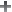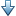## Variance Goal

### Purpose

Limit white-noise impact on specified output signals, when using Control System Tuner.

### Description

Variance Goal imposes a noise attenuation constraint that limits the impact on specified output signals of white noise applied at specified inputs. The noise attenuation is measured by the ratio of the noise variance to the output variance.

For stochastic inputs with a nonuniform spectrum (colored noise), use Weighted Variance Goal instead.

#### Creation

In the Tuning tab of Control System Tuner, select New Goal > Signal variance attenuation to create a Variance Goal.

#### Command-Line Equivalent

When tuning control systems at the command line, use `TuningGoal.Variance` to specify a constraint on noise amplification.

### I/O Transfer Selection

Use this section of the dialog box to specify noise input locations and response outputs. Also specify any locations at which to open loops for evaluating the tuning goal.

• Specify stochastic inputs

Select one or more signal locations in your model as noise inputs. To constrain a SISO response, select a single-valued input signal. For example, to constrain the gain from a location named `'u'` to a location named `'y'`, clickAdd signal to list and select `'u'`. To constrain the noise amplification of a MIMO response, select multiple signals or a vector-valued signal.

• Specify stochastic outputs

Select one or more signal locations in your model as outputs for computing response to the noise inputs. To constrain a SISO response, select a single-valued output signal. For example, to constrain the gain from a location named `'u'` to a location named `'y'`, clickAdd signal to list and select `'y'`. To constrain the noise amplification of a MIMO response, select multiple signals or a vector-valued signal.

• Compute output variance with the following loops open

Select one or more signal locations in your model at which to open a feedback loop for the purpose of evaluating this tuning goal. The tuning goal is evaluated against the open-loop configuration created by opening feedback loops at the locations you identify. For example, to evaluate the tuning goal with an opening at a location named `'x'`, clickAdd signal to list and select `'x'`.

Tip

To highlight any selected signal in the Simulink® model, click. To remove a signal from the input or output list, click. When you have selected multiple signals, you can reorder them usingand. For more information on how to specify signal locations for a tuning goal, see Specify Goals for Interactive Tuning.

### Options

Use this section of the dialog box to specify additional characteristics of the variance goal.

• Attenuate input variance by a factor

Enter the desired noise attenuation from the specified inputs to outputs. This value specifies the maximum ratio of noise variance to output variance.

When you tune a control system in discrete time, this requirement assumes that the physical plant and noise process are continuous, and interprets the desired noise attenuation as a bound on the continuous-time H2 norm. This assumption ensures that continuous-time and discrete-time tuning give consistent results. If the plant and noise processes are truly discrete, and you want to bound the discrete-time H2 norm instead, divide the desired attenuation value by $\sqrt{{T}_{s}}$, where Ts is the sample time of the model you are tuning.

When this option is set to `No`, the closed-loop transfer function being constrained is not scaled for relative signal amplitudes. When the choice of units results in a mix of small and large signals, using an unscaled transfer function can lead to poor tuning results. Set the option to `Yes` to provide the relative amplitudes of the input signals and output signals of your transfer function.

For example, suppose the tuning goal constrains a 2-input, 2-output transfer function. Suppose further that second input signal to the transfer function tends to be about 100 times greater than the first signal. In that case, select `Yes` and enter `[1,100]` in the Amplitude of input signals text box.

Adjusting signal amplitude causes the tuning goal to be evaluated on the scaled transfer function Do–1T(s)Di, where T(s) is the unscaled transfer function. Do and Di are diagonal matrices with the Amplitude of output signals and Amplitude of input signals values on the diagonal, respectively.

• Apply goal to

Use this option when tuning multiple models at once, such as an array of models obtained by linearizing a Simulink model at different operating points or block-parameter values. By default, active tuning goals are enforced for all models. To enforce a tuning requirement for a subset of models in an array, select Only Models. Then, enter the array indices of the models for which the goal is enforced. For example, suppose you want to apply the tuning goal to the second, third, and fourth models in a model array. To restrict enforcement of the requirement, enter `2:4` in the Only Models text box.

### Tips

• When you use this requirement to tune a control system, Control System Tuner attempts to enforce zero feedthrough (D = 0) on the transfer that the requirement constrains. Zero feedthrough is imposed because the H2 norm, and therefore the value of the tuning goal (see Algorithms), is infinite for continuous-time systems with nonzero feedthrough.

Control System Tuner enforces zero feedthrough by fixing to zero all tunable parameters that contribute to the feedthrough term. Control System Tuner returns an error when fixing these tunable parameters is insufficient to enforce zero feedthrough. In such cases, you must modify the requirement or the control structure, or manually fix some tunable parameters of your system to values that eliminate the feedthrough term.

When the constrained transfer function has several tunable blocks in series, the software’s approach of zeroing all parameters that contribute to the overall feedthrough might be conservative. In that case, it is sufficient to zero the feedthrough term of one of the blocks. If you want to control which block has feedthrough fixed to zero, you can manually fix the feedthrough of the tuned block of your choice.

To fix parameters of tunable blocks to specified values, see View and Change Block Parameterization in Control System Tuner.

• This tuning goal also imposes an implicit stability constraint on the closed-loop transfer function between the specified inputs to outputs, evaluated with loops opened at the specified loop-opening locations. The dynamics affected by this implicit constraint are the stabilized dynamics for this tuning goal. The Minimum decay rate and Maximum natural frequency tuning options control the lower and upper bounds on these implicitly constrained dynamics. If the optimization fails to meet the default bounds, or if the default bounds conflict with other requirements, on the Tuning tab, use Tuning Options to change the defaults.

### Algorithms

When you tune a control system, the software converts each tuning goal into a normalized scalar value f(x). Here, x is the vector of free (tunable) parameters in the control system. The software then adjusts the parameter values to minimize f(x) or to drive f(x) below 1 if the tuning goal is a hard constraint.

For Variance Goal, f(x) is given by:

`$f\left(x\right)={‖\text{Attenuation}\cdot T\left(s,x\right)‖}_{2}.$`

T(s,x) is the closed-loop transfer function from `Input` to `Output`. ${‖\text{\hspace{0.17em}}\cdot \text{\hspace{0.17em}}‖}_{2}$ denotes the H2 norm (see `norm`).

For tuning discrete-time control systems, f(x) is given by:

`$f\left(x\right)={‖\frac{\text{Attenuation}}{\sqrt{{T}_{s}}}T\left(z,x\right)‖}_{2}.$`

Ts is the sample time of the discrete-time transfer function T(z,x).